• ## MATLAB自定义函数

千次阅读 2018-11-07 11:29:46
MATLAB自定义函数
分享一下我老师大神的人工智能教程！零基础，通俗易懂！http://blog.csdn.net/jiangjunshow也欢迎大家转载本篇文章。分享知识，造福人民，实现我们中华民族伟大复兴！                第六章 自訂函數  6.1敘述檔與函數檔MATLAB依執行時之實質型式分為兩種，其一為前面所述之敘述檔( script files)，另一為函數檔(function files)；兩者均可在指令窗中呼叫。但敘述檔中，使用者可將檔案內所含的指令一一地在指令窗下執行，其執行過程中，所發生的變數均為整體性變數。因此執行敘述檔時，只能呼叫其檔案名稱，不能在名稱後面加上任何輸入參數。若要事先設定一些參數初值，辦法之一是執行之初立即設定，例如在工作空間內更動其中之變數值，然後再執行該指令。其二是在檔案中利用指令讀取特定檔案之資料或由鍵盤輸入資料。由鍵盤輸入指令可以累積成為指令之組合，並給予指令群之名稱，此名稱因而亦可作為指令在指令窗中直接執行。例如，有一個敘述檔之名稱為 pick_a _number.m，則在 MATLAB 指令窗中直接鍵入"pick_a _number"即可執行該檔案之內容。% pick_a_number.m, a script file for demonstration.% Pick up a number that equals to the randomly generated one% message='Please pick a number from 0-9 ==> ';n=1;while 1    A=input(message);    if isempty(A), A=0; end;    pick=fix(rand*10);    if A==pick,        disp('Congradulations, it''s done!')        break    else        disp(['The random number is ', num2str(pick),...            '. You have failed ',num2str(n),' times!'])        n=n+1;    endend執行的結果如下:>> pick_a_number   Please pick a number from 0-9 ==> 2   The random number is 6. You have failed 1 times!   Please pick a number from 0-9 ==> 3   The random number is 2. You have failed 2 times!   Please pick a number from 0-9 ==> 4   Congradulations, it's done!上述的程式為一個敘述檔，其內容為讀者自訂一個數字，由程式隨機產生一個數字，直到兩個數字相同為止。此程式使用無窮迴圈while 1,…end，故除非答對，才利用break中斷跳出，否則會繼續進行。有關迴圈指令，將在另節詳加討論。在敘述檔中之變數是整體性（global） 的，也是共用的 ，故任何先前定義的變數，均可為敘述檔執行時所用，或改變其內容，所以它不需考慮輸入及輸出之問題。敘述檔用於必須重覆多次使用一群指令時最為方便。函數檔案的內容與敘述檔大略相同，但其檔案可以作為MATLAB語言之一部份，可有輸入及輸出，且其變數的空間是自主的，不與公共空間共用。在型式上函數檔案之開頭一行需有一個函數名稱之宣告，然後用小括符包括其需要之輸入參數。函數名稱前可以有等號，在此等號之左方為其輸出之參數。等號之右方為函數名稱，其後有輸入參數。這些參數變數，無論是輸入或輸出，均可能代表一個數值或一個矩陣。在其他程式語言中，矩陣之變數均需經過宣告，在MATLAB的世界裡，可以省去此項運作，但若屬於大矩陣，事先安排矩陣空間，對提高運算效率有相當的助益。輸出入之參數中，均會預設為矩陣的型式。在函數檔案中，其所屬之變數均屬區域性的，除非特別另宣告為整體性參數。相關的函數特性等後段再作論述。函數檔案之類型純為文字檔，故可在任何文書處理軟體中編輯或修改。不過，MATLAB也有一個程式編輯器，可以在此編輯器中偵錯(Debug)或編輯，這個編輯器比其他文書處理具有特殊的功能，新版中並有行號。若不使用編輯器，也可以在指令窗中亦直接打入type之指令，以觀察該檔案之內容。 張貼者： Martin Fon 位於 10/25/2006 11:20:00 下午 0 意見此文章的連結 標籤： Chap6  6.2 自訂函數之型式 在MATLAB中，可以輕而易舉地定義自己需要之函數，這點大大增強MATLAB之應用能力。一個自定的函數為一個M-檔案，其儲存名稱須與函數名稱相同，格式如下：function [輸出變數]=Name_of_function(輸入參數)函數的名稱需以英文字母開頭，中間可為數字或底線，但其間不能有+,-,*,/等字眼。函數名稱長度依一般檔案名稱之規定，但最多僅認定63個字，不過長度如此長也不盡實際。且其名稱不要與現有MATLAB內定的相同，通常可用isvarname這個函數先檢查。輸出及輸入參數可為多個，亦可為矩陣，但輸入參數必須用一般括號括起來，而輸出參數若僅有一項輸出，則不必用任何括號，若有兩項以上，則需用中括號括起來。下面為一個簡單的例子：function y = freebody(time)% calculation of height with respect to time% time: the elapsed time in seconds%    y:the depth of falling, cm% Example:  yy=freebody(1:20)y =1/2*980*time.*time;％y=(1/2)gt^2這是一個計算自由落體的例子。freebody為函數之名稱，time為其輸入變數，可為矩陣或向量。這個函數以time=[0:4:20]，即time=[0 4 8 12 16 20]代入，執行如下：>> yy=freebody(0:4:20)  yy =0        7840       31360       70560      125440      196000特別注意的是四秒時，自由落體將降至78.4m；若為4.5秒時，約達100m。９１１時紐約世貿大樓幾乎是以這個自由落體的速度塌下來，沒有任何阻檔，所以一直是個謎。在函數內容中，其說明行之前以%開頭，其後面的資料程式即認為是說明，不加執行。依照慣例，函數除第一行外，其最開始不包括空行的幾行為說明行，但每行前面需加%符號。這個說明行主要在敘述該函數之功能及使用方法。當你使用help freebody查詢這個自設的函數指令時，會出現在其說明之內容。例如：>> help freebody time: the elapsed time in seconds    y:the depth of falling, cm Example:  yy=freebody(1:20)程式本體則包括其他函數呼叫、程式結構流程及交談式輸出入、計算、設定指令、註解及空行等。註解可佔全行，亦可在敘述指令之前後。但其前面均需加上%。MATLAB發現有%存在時，其後面之敘述均視為註解。若說明行不需佔有全行，則亦可在指令後加%後，隨時作進一步的說明，其情況如下：　　　y =1/2*980*time.*time; ％y=(1/2)gt^2若有多行的註解行，但不想每行使用%為開頭時，可以在註解之前後行加上%{及%}，這兩個符號必須單獨佔行。此外，MATLAB亦不處理空行，所以為使程式容易閱讀，可以加空行。但在程式開頭的第一次空行會中斷help指令所顯示的內容。函數中所用之變數若不在函數之參數行列出，則應屬區域性變數。若要成為整體性變數則需要特別宣告(如global x y)。下面為兩連桿相接之位置的計算，在此自訂函數two_vectors中，其輸入項為r1,r2,θ1,θ2，輸出為結果點Ｂ之位置(x, y)。程式中，另有自創變數d2r,　th1,　th2等，這些均為區域性變數，故在運算結束跳離函數之後，這些變數將與函數外之參數無關。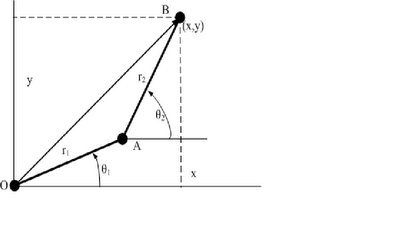function [x,y] = two_vectors(r1,r2,theta1,theta2)% Find the resultant position of two vectors% r1,r2: lengths of the two vectors, cm% theta1, theat2: horizontal angles of r1 & r2, deg.%  x,y:coordinates of the tip position.cm% Example:  [xx,yy]=two_vectors(5,8,30,60)d2r=pi/180;th1=theta1*d2r;th2=theta2*d2r;x =r1.*cos(th1)+r2.*cos(th2);y =r1.*sin(th1)+r2.*sin(th2);上項例子設兩桿之長度為5cm及8cm，對應水平角為20及50度，則經執行指令之結果如下：>> [xx,yy]=two_vectors(5,8,20,50)xx =    9.8408yy =    7.8385 張貼者： Martin Fon 位於 10/25/2006 11:10:00 下午 1 意見此文章的連結 標籤： Chap6  6.3 函數之編輯 前面所設之函數名稱如freebody、two_vectors等在存檔時，必須直接以函數名稱為其檔案名。以後呼叫時才能找到這個檔案來執行。存檔時，必須以這兩檔的名稱加上”.m”的字尾，即如freebody.m、two_vectors.m。撰寫這些函數檔可用MATLAB的編輯器(editor)，只要從檔案指令下開檔即可找到。或直接在指令窗下這樣的指令：>>edit two_vectors.m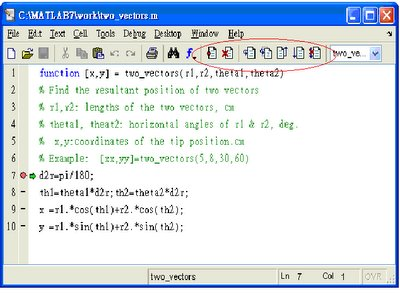編輯器可同時開啟許多檔，但僅能執行其中一個檔。編輯窗具有行號，可以進行偵錯及修改，相當方便。作為註解的部份則會以不同的顏色顯示，只有真正程式內容部份用黑色（預設值），但其行號仍然由第一行算起。在偵錯期間，此行號成為指向錯誤點的定位方法。編修完後的檔案即可存成.m檔。進行偵錯時，可以採用單步法或全程執行模式，但可設中斷點。只要用滑鼠到行號右側一點，該會顯示一紅點。將來程式執行時，抵達中斷點會暫時停止。此時可以利用鍵盤下各種指令以確定執行過程中各項變數的變化。中斷點可以設好幾點，執行時會依序暫停。要解除中斷點，只要重複用滑鼠再選一次即可，或指向該行然後在指令行上按打叉的指令即可。單步法選下圖第三項，每按一次僅執行一行，如此可以偵錯全部。第四項與第三項相同，但可進行呼叫的函數內部逐步執行。第六項為往前執行，直接終了，或遇到紅點的行為止。其餘讀者可以自行試驗其功能。MATLAB的新功能中，有一項是可以檢驗所選寫程式的執行效率。這個功能置於編輯器的工具項中的一個選項，稱為M-LINT檢驗程式碼(Check code with M-Lint)。這個選項可以在程式完成偵錯後，再進一步檢查程式的執行效率。由此可以得到一個建議的報告(M-Lint Code Checker Report)，其內容有相關修正事項，並如何使程式執行時更有效率，且節省處理時間等等。對於大程式而言，這項功能相當有用。張貼者： Martin Fon 位於 10/25/2006 11:00:00 下午 2 意見此文章的連結 標籤： Chap6  6.4次函數 一個函數檔案可以包含一個以上的函數，這些多餘的函數又稱為次函數或副函數(Subfunction)。這些副函數僅能為主函數或其他同檔案之次函數相互呼叫。每個次函數均有其函數之定義行，而且一個接一個置於主函數後面，其順序不拘。下面為一個畫圓的例子，輸入圓的半徑，然後以亂數函數決定其圓心位置，再利用plot指令繪出圓。程式先從drawcircles(r)進入，在其for…end之迴圈中呼叫次函數circ(rr)決定圓心位置及計算圓的座標，而在其中又呼叫randxy之次函數，計算圓心位置，此時即為次函數呼叫次函數之例子。在主函數中利用plot指令則只要將對應之X、Y座標值輸入就可，其結果如圖。function drawcircles(r) % 主函數% Draw circles with radii of r.global MAXR;n=length(r);MAXR=max(r);hold on;for i=1:n,   [X,Y]=circ(r(i));   plot(X,Y);endhold off;axis equal;endfunction [x,y]=circ(rr)%次函數% Calculate the points of a circle.   [xx,yy]=randxy;   theta=linspace(0,2*pi,60);   x=xx+rr*cos(theta);   y=yy+rr*sin(theta);endfunction [xx,yy]=randxy%次函數global MAXR% locate the position of the center.xx=rand*MAXR;yy=rand*MAXR;end執行此程式：drawcircles(2:10)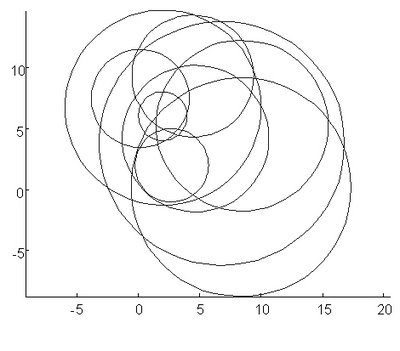由於使用亂數決定各圓之圓心，故每次執行上項程式均會有不同的結果。只是其半徑均會一樣，本例之半徑為[4 3 1 5]。程式中，除兩個次函數circ 與randxy外，尚有呼叫max及length兩函數，這些都是MATLAB的內在函數。次函數內之變數必須透過輸出入參數傳遞，否則僅能在自家之函數範圍內使用，包括主函數及其他次函數均無法讀取該次函數內之變數。除非將要共用之變數宣告為全域性(Global)。例如，函數中有用到全域變數MAXR，必須在使用到的函數（如randxy）中要宣告，才能共同使用。呼叫次函數時，MATLAB首先檢查是否為次函數，然後檢查具有相同名稱之自有函數，最後才是內建函數。故若有相同函數名稱仍以次函數為優先。 張貼者： Martin Fon 位於 10/25/2006 10:50:00 下午 0 意見此文章的連結 標籤： Chap6  6.5匿名函數 匿名函數是一種不必具有函數檔的型式即可進行呼叫的函數。因此建立匿名函數可以在敘述檔或函數檔中加入，甚至在指令窗內加入即可。其基本型式如：fhandle=@(arglist) expression這個型式右邊以＠開始，利用這個符號產生函數之握把(handle)，以此作為呼叫此函數之起始，形成匿名函數之一部份。括號內之arglist為自變數名單，是由外進入函數之變數。而經空一格後之expression則為函數之主體。此匿名函數之握把可以設定給左邊之變數fhandle，利用此變數呼叫此匿名函數。下面為例子：>>circ=@(r) r.*r*pi;>>circ(2:2:10)ans = 12.5664   50.2655  113.0973  201.0619  314.1593這是一個計算圓面積的例子，只要輸入半徑，即可得到圓的面積。這時之自變數為r，而函數握把為circ，也是匿名函數之名稱。利用此握把，即可下指令執行該函數，因此直接將其作為函數之名稱餵入參數項即可執行。例如計算一個圓環所佔的面積，設內環半徑為2、外環為4，則以LoopArea可得：>>LoopArea=circ(4)-circ(2)>>LoopArea = 37.6991若為兩個自變數，則其變數可以酌增，如：>>Cllipse=@(x, y)   3*x.*x + 5*y.*y;>>Cllipse([3 4 6],[5 7 8])ans = 152   293   428利用匿名函數，以小型函數為主，故使用上相當方便。這種函數亦可分組在細胞陣列之中，隨時呼叫所需進行之計算，例如：>>Afun={@(x) x.*x,  @(y) y.*y+100 , @(x,y) x.*x + y.*y + 100}Afun =Columns 1 through 2  [@(x) x.*x]    [@(y) y.*y+100]Column 3  [@(x,y) x.*x + y.*y + 100]>>Afun{1}(5) + Afun{2}(10)ans =   225>>Afun{3}(5,10)ans =   225由於所設的匿名函數值第三項等於第一及第二項之和，故上述所求得之結果應會相同。匿名函數定義時，其內也可以使用常數。但這些常數必須事先定義，才能完成匿名函數的型式。此匿名函數之常數將維持原先設定之值，不再改變。故若在運算過程中間即使更改該常數值，也不更動匿名函數之原常數值，除非重新定義該匿名函數。以下為例：>>a=2;b=3; f=@(x) (a*x+b)>>f =     @(x) (a*x+b)>>f(2)ans =     7>>a=5;b=8;f(2)ans =     7顯然這個匿名函數並未因常數a與b之改變而改變。必須必須在常數改變之後重新定義才能生效：>>a=5;b=8; f=@(x) (a*x+b)f =     @(x) (a*x+b)>>f(2)ans =    18在MATLAB有繪圖指令fplot可以繪製一般函數，其輸入只要函數及其前後之範圍：>>a=3;b=4;g=@(x) (a*x.^2 +b*x)g =     @(x) (a*x.^2 +b*x)>>fplot(g, [-10 10])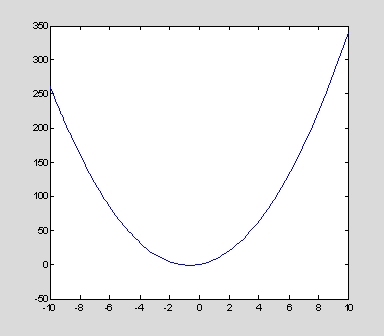也可以直接將匿名函數整個內容置入於其第一參數之中：>>fplot(@(x) (a*x.^2 + b*x), [-20 20])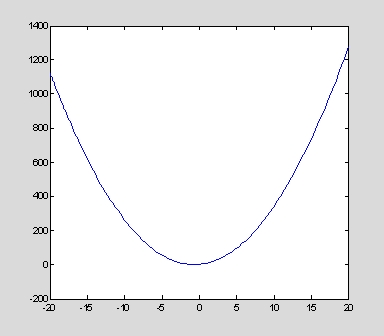張貼者： Martin Fon 位於 10/25/2006 10:40:00 下午 0 意見此文章的連結 標籤： Chap6  6.6巢狀函數 在函數中又存在另一個函數，這是MATLAB函數中基本的型態，不過大部份是另一個函數附於主函數之下，因此可以隨時呼叫。但此處所謂巢狀函數(Nested function)是處於函數內文之中，或以自己為函數重新呼叫。撰寫一個巢狀函數與一般函數相同，亦即在函數中鉗置一個格式相同的函數：function mainfun=A(p1, p2)%主函數  E(p1); B(p1); D(p2);A(p1,p2)  function infun1=B(p3)　%第一層巢函數      C(p3);E(p3);B(p3);D(p3);A(p3,p3);      function infun1=C(p4)　%第二層巢函數　　　　   C(p4);E(p4);B(p4);D(p4);A(p4);　　　　end  end  function infun1=D(p3)　%第一層巢函數  　  F(p3);E(p3);B(p3);D(p3);      function infun1=F(p4)　%第二層巢函數　　　　  F(p4);E(p4);B(p4);D(p4);A(p4,p4);　　　　end  end  ---endfunction main2=E(p5) %次函數E(p5); A(p5,p5);end一般函數並不需要在終了加一個end，但若要做成巢狀結構，則必須以end結束。中間之函數可以再包含另一個巢狀函數，或者最外圍亦可包含多個巢狀函數。在呼叫層次則有限制。第一層主函數可以呼叫次函數E及其下之第二層巢B與D函數，但不能呼叫其下之第三層以下如Ｃ函數。低層函數則可以呼叫其本身及同層以上之高層函數（如Ｄ可呼叫Ｄ、Ａ、Ｂ、Ｅ等函數。函數中之變數影響範圍則依其巢函數之位置而定。通常主函數與次函數各享有自己的工作空間，故除非利用輸入參數傳介，或宣告全域性變數，否則這兩個工作空間是相互獨立的。巢函數則與次函數不同，因為它與其直屬函數共享有工作空間。因此，巢函數本身雖有自己的獨立的空間，但由直屬函數內之變數則是共用的，因此這些變數均可能在兩個函數裡被更改。巢函數置於某一函數之中，在執行時除非經由呼叫程序，否則不會自動執行。因為它也具有閉鎖性質，其變數也需經由輸出入參數傳輸。下面為一個計算多項式值的函數nest_fun。其輸入x 值可以為列矩陣，而係數a有三項，分別為A、B、C ，a可以有多項輸入。如此計算可以得到不同的組合。本函數利用細胞陣列rr_arry作為儲存運算結果，讀者可試著瞭解前節所介紹的功能。要顯示其內容必須使用celldisp指令。function [rr_array]=nest_fun(x,a)%function to find sets of polynormials.% a: set of constants, [A B C]% x: variables in array% Example: rr=nest_fun(2:10,[1 2 4;2 4 8])n=size(a);for i=1:n  A=a(i,1);B=a(i,2);C=a(i,3);  rr_array{1,i}=['A=',num2str(A),', B=',...      num2str(B),', C=',num2str(C)];  rr_array{2,i}=polyx(x);　end  function r=polyx(xx)  　　r=A.*x.^2 + B.*x +C;  endend執行例：>> rr=nest_fun(2:10,[1 2 4;2 4 8]) rr =  'A=1, B=2, C=4'    'A=2, B=4, C=8'     [1x9 double]       [1x9 double]>> celldisp(rr)rr{1,1} =A=1, B=2, C=4rr{2,1} =    12    19    28    39    52    67    84   103   124rr{1,2} =A=2, B=4, C=8rr{2,2} =    24    38    56    78   104   134   168   206   248 張貼者： Martin Fon 位於 10/25/2006 10:30:00 下午 0 意見此文章的連結 標籤： Chap6  6.7函數輸出入參數 在函數應用中，變數之轉換至為重要。一般函數內定義之變數均屬區域性變數(Local variables)，只能提供該函數內部使用，當函數執行完畢後，即被清除。為維持參數之一貫性，當呼叫某函數，也必須同時傳遞重要之變數作為該函數之輸入值，以為繼續計算之根據。執行函數完畢時，有些結果仍寄望能傳回呼叫主程式，此時必須有輸出參數。6.7.1全域性變數有些變數對許多函數而言有時同等重要，此時就可宣告為全域性變數(Global variables)，並在需要此變數的函數中同時宣告。此變數即可共同使用，不會因函數之轉換而遭到清除。在前面所討論的敘述檔中，實際上其所使用的變數均為全域性的變數。因此每次執行後，其相關之變數均有可能被更改。全域性變數之宣告如下例：global GRAVITY X Y Z上式即宣告GRAVITY、X 、Y、Z等四個變數為全域性，習慣上均用大寫，以與其他區域型變數區別。不過，這並不是必然的作法，小寫也一樣可用。6.7.2 eval函數一般下指令時，通常以其指令名稱及需要之參數在指令窗下或在檔案中直接下，但有些時候我們希望指令也是一種變數的型式，使指令內容也能依檔案之內容而變化。換言之，先將指令化為文字串，但然依文字串之內容轉為指令去執行。MATLAB提供一個eval函數具有轉換的功能，其格式如下：eval(指令相關敘述)例如：以下面程式求某一連續正整數累進之總和% evalsum.m for demonstration% Find the sum of cumulative sum(1:n)tsum=zeros(1,10);xsum=0;for i=1:10,   xsum=xsum+eval(['sum(1:',int2str(i),')']);   tsum(i)=xsum;end1:10tsum執行後：>> evalsum   ans =     1     2     3     4     5     6     7     8     9    10    tsum =   1     4    10    20    35    56    84   120   165   220式中之eval函數是將sum(1:1)、sum(1:2),…sum(1:10)逐一計算後累加。由於其中sum之參數逐一變化，故必須用數值改變為字串後(int2str)，再用eval函數轉為指令執行之。6.7.3 輸出入參數個數nargin與nargout呼叫函數時，大部份需要輸入參數，經過處理過後，也需要輸出參數以獲得希望的結果。為使程式能掌握呼叫的參數，通常程式中有設定輸入與輸出參數之個數。其變數分別為nargin與narout，使程序進行前能依實際需要，決定所要進行的步驟。所以上述兩參數名稱已為程式內部參數，程式中可依需要使用，但在設定變數時，需不得重覆。下面的例子為計算空心圓柱體體積之程式，主要針對輸入項少於原設值時，採用預設值的方式。function [volume]=pillar(Do,Di,height)% Find the volume of a hollow pillar% ro,ri:outside & inside diametersif nargin<1,    height=1;    di=0;   do=1;endvolume=abs(Do.^2-Di.^2).*height*pi/4;此程式利用nargin變數，確定所缺少的輸入項並補上預設值。所以即使不使用輸入參數，其結果將以直徑及高度均為一單位之圓柱體計算其體積。如：>>pillar  ans =   0.7854 >>pillar(5,2,[1:5])  ans =  16.4934   32.9867   49.4801   65.9734   82.4668 即使利用pillar函數，亦可計算陣列輸入時之對應體積。可以增加程式之使用彈性。對於nargout參數之應用亦與nargin相同，當下指令時，有時未提出輸出的項目，此時其nargout=0。此時亦可利用這種情況決定是否進行繪圖或作其他動作。 張貼者： Martin Fon 位於 10/25/2006 10:20:00 下午 0 意見此文章的連結 標籤： Chap6  6.8私有目錄函數 在MATLAB的目錄中，有一個private子目錄，任何函數置於此目錄中，可以獲得優先執行。由母目錄可以事先看到其中的函數，因此主函數也可以利用此目錄之區隔獲得執行之優先權。為此若有兩相同名稱之m-檔案，一若置於private子目錄而另一置於其他目錄。則在private子目錄則在下之同名稱檔案會優先被執行，置於其他目錄檔案者雖然名稱相同，亦不會被執行。private子目錄通常無法用檔案總管觀察，但可以用下列指令得到訊息：>>help private/myprivfun張貼者： Martin Fon 位於 10/25/2006 10:05:00 下午 0 意見此文章的連結 標籤： Chap6  6.9函數為輸入參數之呼叫法 有些函數之輸入參數必須呼叫其他函數名稱，以該函數進行運算。例如fzero、fminsearch、 fminbnd等等，其中均需輸入或呼叫適當函數名稱，使運算正常進行。大體上傳輸函數之方法有四：一、 以字串之型式對照Ｍ函數檔之名稱。例如：function y = freebody(time)% calculation of height with respect to time  y =1/2*980*time.*time;  >>[x,f]=fzero('freebody',[0 1])  x =  0  f =  0二、 以現存函數名稱之握把呼叫，例如：  >>[x,f]=fzero(@freebody,[0 1])       x =     0     f =     0或  >>f1=@freebody;[x,f]=fzero(f1,[0 1])     x =     0    f =     0三、 利用inline指令轉換，例如：　　>> g=inline('freebody(time)')     g =     Inline function:     g(time) = freebody(time)  >> [x, value]=fzero(g,[0 1])     x =     0     value =     0四、 直接使用運算式，例如：   >>f1='x.^2-5';[x, value]=fzero(f1,[0 5])     x =    2.2361     value =  8.8818e-016或，    >>[x, value]=fzero('x.^2-5',[0 5])       x =    2.2361      value =  8.8818e-016上述四種呼叫方式原則上均可適用。但在MATLAB之應用上，仍以握把呼叫較有效率，其執行速度也較快。雖然如此，由於撰寫程式本乎於習慣，故何者為佳，端視當時情況而定。一般之匿函數屬於握把呼叫型，可以使用在任何主函數內的位置。但這種函數以簡短為主，無法應用在大程式上。以下面之三個匿名函數為例，f1、f2各自定義內容，而g則由f2配合f1，輾轉以x為自變數，則不必利用傳統的函數型式，可以輕易加以串聯，並得到g([1 2 3])之結果：>>f1=@(x) sqrt(x);>>f2=@(x) 5*exp(-x);>>g=@(x) f2(f1(x));>>g([1:3])  ans =  1.8394    1.2156    0.8846            给我老师的人工智能教程打call！http://blog.csdn.net/jiangjunshow展开全文• Matlab中关于自定义函数的教程，专业课时的教师讲义PPT
• Matlab 自定义函数的五种方式 命令文件/函数文件+ 函数文件 － 多个 M 文件 函数文件 + 子函数 － 一个 M 文件 inline － 无需 M 文件 syms + subs 方式 － 无需 M 文件 字符串 + subs 方式 － 无需 M 文件
• 本书主要是讲MATLAB自定义函数，适合初学者使用！
• matlab自定义函数（滤波器部分），dsp运用到的函数，，AFD_BUTT.M，AFD_CHB1.M，AFD_CHB2.M，AFD_ELIP.M，BLACKMAN.M，CAS2DIR.M，CASFILTR.M等等
• 实验5 Matlab 自定义...1. 学习Matlab自定义函数命令及求最小值命令;例1;function y=f1(x) y=x^2+sin(x)+2;Matlab 求最小值命令 fmin 调用格式: ;例1;若想画出标准正态分布的密度函数的图像则输入;例2;[r1 r2]=rootq
• matlab自定义函数和导数应用的简单介绍和实际例程。
• matlab自定义函数创建与使用 [自定义函数创建] (https://zhinan.sogou.com/guide/detail/?id=316513514107) 　可以先看下这个链接内容，写这个内容，提醒我记住遇到的问题，另外如果大家有这方面的问题的话，也可以...
matlab自定义函数创建与使用
[自定义函数创建]
(https://zhinan.sogou.com/guide/detail/?id=316513514107)
可以先看下这个链接内容，写这个内容，提醒我记住遇到的问题，另外如果大家有这方面的问题的话，也可以帮助解决，不要总把使用VS在编写C语言的想法，带到matlab中，也一定要坚持找到自己的问题，MATLAB函数创建在一个单独M文件中，图片是函数文件第一行，在调用只要保证与调用它的的主函数在一个文件夹中，在主函数中定义输入变量，方可调用，接着输入  [输出变量 ]函数名（输入变量）可计算出输出变量的值。图片是我在定义好输入变量之后，调用时输的内容。展开全文• 自定义函数，tstem(hn,y)，可以用来作离散序列柄状图。
• MATLAB在学习生活中应用非常广，简单总结一点MATLAB的使用小技巧，在脚本中自定义函数表达式并进行绘制 主要包括： 线性： f(x)=ax+b 二次：f(x)=ax^2+bx+c 高斯函数： 广义高斯函数: 对数正态函数: 代码实现的...
MATLAB在学习生活中应用非常广，简单总结一点MATLAB的使用小技巧，在脚本中自定义函数表达式并进行绘制
主要包括：

线性： f(x)=ax+b
二次：f(x)=ax^2+bx+c
高斯函数：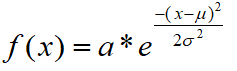广义高斯函数: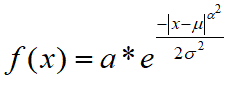对数正态函数: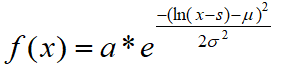代码实现的主要思路为：

使用了syms函数来定义变量
自定义函数表达式
定义自变量x的取值范围
使用subs函数把x的值代入自定义好的表达式中，求解出因变量的值
使用plot函数绘制自定义函数的曲线

绘制曲线图为：
线性函数和二次函数：
函数表达式为：fx1 = x + 5 以及 fx2 = x^2 + 1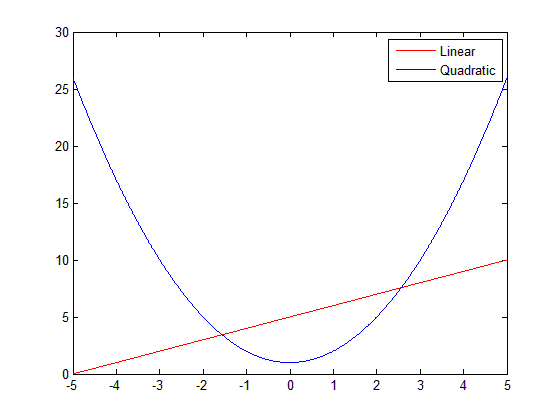高斯函数与广义高斯函数：

注意：alpha的取值决定了函数顶部的尖锐程度，当alpha<sqrt(2)时曲线很窄；alpha=sqrt(2)时为标准的高斯函数；alpha>sqrt(2)时，曲线的顶部变宽。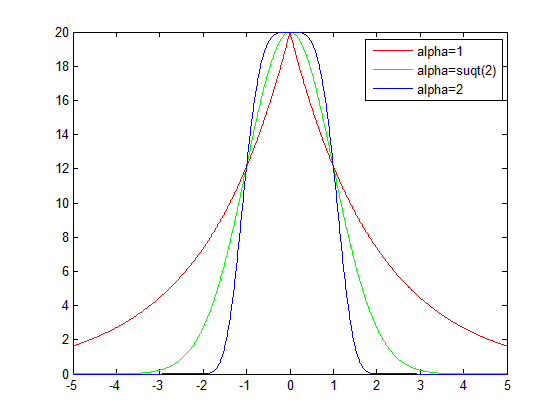对数正态函数：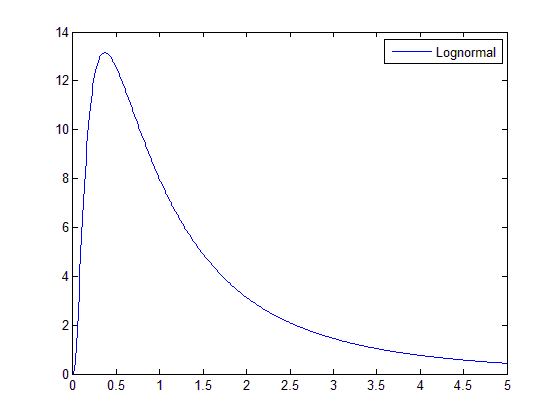源代码如下（若格式乱了也可以通过：此链接下载相关文件）：
%% xiaochen wang 2020/07/11
% plot function curve
% such as:
% linear:f(x)=ax+b
% quadratic:f(x)=ax^2+bx+c
% gaussian function:f(x)=a*exp(-(x-μ)^2/2σ^2)
% generalized gaussian function:f(x)=a*exp(-|x-μ|^α^2/2σ^2)
% lognormal function:f(x)=a*exp(-(ln(x-s)-μ)^2/2σ^2)

clc;
clear all;

syms x fx1 fx2 fx3 fx4_1 fx4_2 fx5; % creating symbolic variables or function

a = 20; miu = 0; xigma = 1; % define the parameters of gaussian function, A,μ，σ
alpha = sqrt(4); % define the value of parameter α (generalized gaussian function)
s = 1; % define the value of parameter s (lognormal function)

fx1 = x + 5; % linear
fx2 = x^2 + 1; % quadratic
fx3 = a*exp(-(x-miu)^2/(2*xigma^2)); % gaussian
fx4_1 = a*exp(-abs(x-miu)^(alpha^2)/(2*xigma^2)); % generalized gaussian
alpha = sqrt(1); % modify the value of alpha
fx4_2 = a*exp(-abs(x-miu)^(alpha^2)/(2*xigma^2)); % generalized gaussian
fx5 = a*(1/(x*xigma*sqrt(2*pi)))*exp(-(log(x)-miu)^2/(2*xigma^2)); %1/x*xigma*sqrt(2*pi)

xx = -5:0.01:5; %variables x
xx2 = 0.01:0.01:5; %variables x in lognormal function must greater than zero
ans1 = subs(fx1, x, xx);
ans2 = subs(fx2, x, xx);
ans3 = subs(fx3, x, xx);
ans4_1 = subs(fx4_1, x, xx);
ans4_2 = subs(fx4_2, x, xx);
ans5 = subs(fx5, x, xx2);

figure('Name', 'Linear_and_Quadratic');
plot(xx, ans1, 'r'); % plot linear function
hold on;
plot(xx, ans2, 'b'); % plot quadratic function
legend('Linear', 'Quadratic');

figure('Name', 'Gaussian_and_Generalized gaussian');
plot(xx, ans4_2, 'r'); % alpha = 1
hold on;
plot(xx, ans3, 'g'); % alpha = sqrt(2)
hold on;
plot(xx, ans4_1, 'b'); % alpha = 2
legend('alpha=1', 'alpha=suqt(2)', 'alpha=2')

figure('Name', 'Lognormal');
plot(xx2, ans5);
legend('Lognormal');



展开全文• Matlab自定义函数的几种方法 1、函数文件+调用命令文件：需单独定义一个自定义函数的M文件; 2、函数文件+子函数：定义一个具有多个自定义函数的M文件； 3、Inline:无需M文件，直接定义； 4、匿名函数； 5、...
Matlab自定义函数的几种方法

1、函数文件+调用命令文件：需单独定义一个自定义函数的M文件;
2、函数文件+子函数：定义一个具有多个自定义函数的M文件；
3、Inline:无需M文件，直接定义；
4、匿名函数；
5、Syms+subs: 无需M文件,直接定义；
6、字符串+subs：无需M文件,直接定义；
7、直接通过@符号定义.
1、函数文件+调用函数文件：定义多个M文件：
% 调用函数文件:myfile.m
clear
clc
for t=1:10
y=mylfg(t);
fprintf(‘M^(1/3)=%6.4f\n’,t,y);
end
%自定义函数文件: mylfg.m
function y=mylfg(x) %注意：函数名（mylfg）必须与文件名（mylfg.m）一致
Y=x^(1/3);
注：这种方法要求自定义函数必须单独写一个M文件，不能与调用的命令文件写在同一个M文件中。
2、函数文件+子函数：定义一个具有多个子函数的M文件
%命令文件：funtry2.m
function []=funtry2()
for t=1:10
y=lfg2(t)
fprintf(‘M^(1/3)=%6.4f\n’);
End
function y=lfg2(x)
Y= x^(1/3);
%注：自定义函数文件funtry2.m中可以定义多个子函数function。子函数lfg2只能被主函数和主函数中的其他子函数调用。
3、Inline:无需M文件，直接定义；
%inline命令用来定义一个内联函数：f=inline(‘函数表达式’, ‘变量1’,’变量2’,……)。
调用方式：y=f(数值列表) %注意：代入的数值列表顺序应与inline()定义的变量名顺序一致。
例如：
f=inline(‘x^2+y’,’x’,’y’);
z=f(2,3)
Ans=7
注：这种函数定义方式是将它作为一个内部函数调用。特点是，它是基于Matlab的数值运算内核的，所以它的运算速度较快，程序效率更高。缺点是，该方法只能对数值进行代入，不支持符号代入，且对定义后的函数不能进行求导等符号运算。
例：
Clear
Clc
f=’x^2’;
Syms x g;
g=x^2;
h=inline(‘x^2’,’x’);

4. 匿名函数
使用matlab函数句柄操作符@，可以定义指向matlab内置函数和用户自定义函数的函数句柄，函数句柄也可以像函数一样的使用。例如：
>>x=-pi:0.1:pi;
>> fh={@cos,@sin};
fh =@cos @sin
>>plot(fh{2}(x))
5、Syms+subs: 无需M文件,直接定义；
用syms定义一个符号表达式，用subs调用：
Syms f x %定义符号
f=1/(1+x^2); %定义符号表达式
subs(f, ‘x’, 代替x的数值或符号)
注：对于在syms中已经定义过的符号变量，在subs中进行替代时，单引号可以省略。但是，如果在syms后又被重新定义为其他类型，则必须加单引号，否则不可替换。
这种函数定义方法的特点是，可以用符号进行替换
Syms f x
f=1/(1+x^2);
subs(f, ‘x’,’y^2’)
ans=
1/(1+(y^2)^2)
注：该方法的缺点是，由于使用符号运算内核，运算速度会大大降低。
6、字符串+subs：无需M文件,直接定义；
直接定义一个字符串，用subs命令调用。例如：
f=’1/(1+x^2)’ %定义字符串
z=subs(f,’x’,2)
g=subs(f,’x’,’y^2’)
注：优点是，占用内存最少，定义格式方面自由。
缺点是，无法对字符进行符号转化。
当所要替代的符号在调用前都已经有了数值定义，则可以直接调用：subs(f).例如：
f=’x^2*y’;
x=2;y=3;
subs(f)
ans=12
7、直接通过@符号定义.
示例如下：
>> f=@(x,y)(x.^2-sin(y))
f =@(x,y)(x.^2-sin(y))
>> f(2,3)
ans =3.8589


展开全文• 在使用matlab时，常常需要用到自己写的function函数（自定义函数），那么调用自定义函数MATLAB默认的路径是怎么样的？ 实际上，当我们运行m文件时，系统会搜索两个路径下的m文件（自定义函数等）和程序中用到的...
• 函数名：vd 作者：Freeman 日期：2020.5 应用：在笛卡尔坐标系、柱坐标系和球坐标系下计算梯度、散度、旋度与拉普拉斯量 输入： u 被求导函数 v 函数自变量，笛卡尔坐标系下为[x y z]，柱坐标系下为[s phi z]，球...
• ## 实验5matlab自定义函数的编写

万次阅读 多人点赞 2018-09-28 11:11:58
自定义一个函数，求给定复数的指数、对数、正弦和余弦，并在命令文件中调用该函数自定义一个函数，计算一行向量中各元素的均值和标准差。 求下列方程的根： f(z)=&amp;nbsp;ex+x2+xx=100&amp;amp;...
• ## matlab自定义函数的几种方法

万次阅读 多人点赞 2017-10-14 09:20:05
1、函数文件+调用命令文件：需单独定义一个自定义函数的M文件; 2、函数文件+子函数：定义一个具有多个自定义函数的M文件； 3、Inline:无需M文件，直接定义； 4、匿名函数； 5、Syms+subs: 无需M文件,...
• ## MATLAB自定义函数拟合

万次阅读 多人点赞 2017-08-28 17:21:09
%自定义拟合函数f(t)=a*cos(k*t)*exp(w*t) clc,clear syms t x=[0;0.4;1.2;2;2.8;3.6;4.4;5.2;6;7.2;8;9.2;10.4;11.6;12.4;13.6;14.4;15];%列向量 y=[1;0.85;0.29;-0.27;-0.53;-0.4;-0.12;0.17;0.28;0.15;-0.03;-......

# matlab自定义函数matlab 订阅